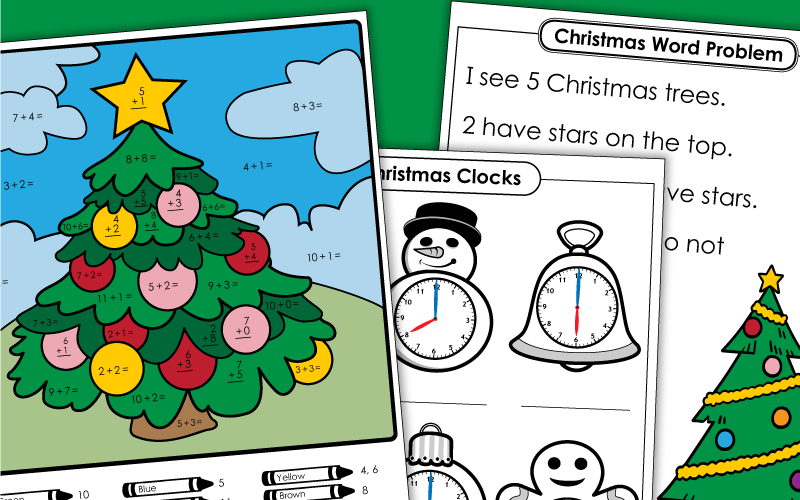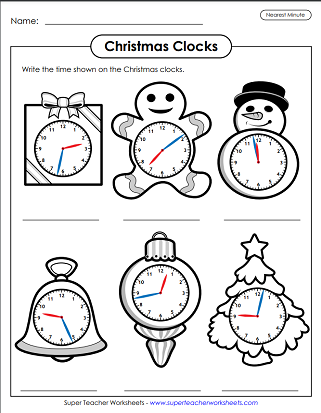# Christmas Math

This page has a collection of Christmas-themed mathematics worksheets that you can print and use with your students.First, write the answers to the addition facts. (Facts have addends up to 10.) Then follow the code at the bottom to color the spaces. The finished product is a portrait of Santa Claus.
Write the answers to the basic addition problems (with addends up to 10). Then color each part of the tree according to the key at the bottom of the page.
Write the sum for the basic addition facts, then color the stocking according to the key.
Write the sums for the basic addition facts, then color according to the key.
Write the sums for the basic addition facts, then color according to the key. The mystery picture is a reindeer.
Solve the 2-digit addition problems; Without regrouping; Christmas tree pictures
Solve the 2-digit addition problems; Requires regrouping; Christmas tree pictures
Add to find the sums. Each problem is presented in a Christmas picture.
Find the sum for each 3-digit addition problem. Then cut out the puzzle pieces. Match the number (sum) on each puzzle piece with the corresponding addition problem. The completed puzzle shows a lovely gingerbread house.
Check the price tags and add the numbers to determine how much you'll spend on each person at the Secret Santa Market.

## Christmas Subtraction

After solving the basic subtraction facts, color each part of the Santa picture as indicated by the key at the bottom.
After writing the answers to basic subtraction problems, students color the Christmas tree according to the color code at the bottom of the page.
Write the difference for each of the basic subtraction facts, then color according to the key.  Christmas stocking picture.
Write the answer for each basic subtraction facts, then color according to the key.
Solve the 2-digit subtraction problems; Requires borrowing; Christmas tree pictures
Solve the 2-digit subtraction problems; Requires borrowing; Christmas tree pictures
Subtract 3-digit numbers. Most problems require regrouping. Each problem is presented inside of a Christmas picture.
Solve the 3-digit subtraction problems. (Most problems require regrouping.) Then cut out the puzzle parts. Match each difference with the correct problem to complete the puzzle. The completed puzzle shows Santa placing gifts below a tree.

## Christmas Multiplication Worksheets

Write the correct product in each space. Then color according to the key on the bottom part of the page. Finished picture is a portrait of Santa Claus.
Find the product for each pair of factors on this Christmas tree illustration. The use the code at the bottom to color the spaces.
Write the product for each of the basic multiplication facts, then color according to the key.  Christmas stocking picture.
Write the product for each of the basic multiplication facts, then color according to the key.  Christmas candle picture.
Write the products for the basic multiplication facts, then color the illustration according to the key. The mystery picture reveals a reindeer.
Complete the missing numbers on the Christmas ornament number bonds. Use multiplication or division to solve each.
Solve the double-digit by single-digit multiplication problems. Then cut out the puzzle pieces. Match each problem with its product. The finish puzzle reveals a holiday fireplace scene. with stockings, presents, and a tree.
Solve the 2-digit by 1-digit multiplication problems; Christmas tree pictures
This worksheet has nine multiplication problems for students to solve.

## Christmas Division Worksheets

Solve the division facts and color the worksheet according to the code at the footer of the worksheet.
Write the quotient for each basic division fact shown. Then apply the coloring code at the bottom to the illustration.
Divide to find the products, then color according to the key to reveal a Christmas stocking.
Write the quotient for the basic division facts, then color according to the key.  Christmas candle picture.
Write the products for the basic multiplication facts, then color the illustration according to the key. The mystery picture reveals a reindeer.
Division problems with 2-digit dividends and remainders; Christmas tree pictures
Solve the division problems. Each one has a 2-digit dividend and a remainder. Then match the puzzle pieces with the quotients. The main version of this worksheet has remainders, but you can click on the "alt" button below if you'd rather have the remainders listed as fractions.

## Graphing

Plot the ordered pairs on the coordinate plane. Then simply connect the dots to create a picture of a Christmas tree.
Plot the ordered pairs and connect the dots to create a portrait of Santa.
Count the different objects on the page and use the numbers to create a bar graph. Then use the graph to answer the questions.

## Christmas Word Problems

This file contains a dozen word problems. Each one requires children to add or subtract.
Students will need to add and subtract money to solve these word problems.
Students will need to have a basic understanding of fractions to solve these word problems.

## Geometry

Your students will use scissors to cut out the polygons and circles. Use them to make a Christmas tree, a snowman, and a Santa hat.

## Christmas ClocksTelling Time

Write the times shown on the Christmas clocks. Times are to the nearest hour. (Examples: 8:00, 12:00, 7:00, etc.)
State the times. Clock are shown with times in 5-minute increments. (examples: 5:15, 3:40, 6:05)
Read the time on each Christmas clock. Times are shown on analog clocks with minute increments. (examples: 2:32, 7:09, 11:58, etc.)

## Calculating Volume

Multiply length × width × height to find the volume of each holiday gift box shown.
Calculate the volumes of the Christmas present boxes. Then add to find the combined, additive volume. Basic level features single-digit whole numbers. All side lengths given.
Students find the volumes of the Christmas presents. Then add to find the additive volume of the composite shape. Intermediate level features single-digit whole numbers. Some side lengths are unknown variables.
Find the volumes of the boxes used for Christmas presents. Then add to find the combined volume. Advanced level features larger numbers and decimals. Calculator recommended for this level.

## Color-by-Number

Complete this color-by-number worksheet to reveal a picture of a waving Santa.

## December Calendars

Here is a printable December calendar. After you print it out, have students number the squares and add important holidays.
Your class will love designing and coloring this snowman calendar.
More Christmas Printables

## Sample Worksheet ImagesMy Account
Site Information